# AP Biology : Understand ATP coupling

## Example Questions

### Example Question #1 : Cellular Energetics

Given the hydrolysis of 1 mole of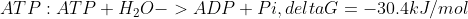, which reaction could be driven by hydrolyzing 2 moles of ATP?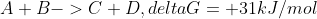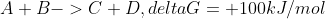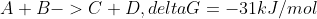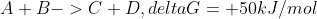Explanation:

Given that the hydrolysis of 1 mole of ATP has delta G of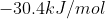, if 2 moles of ATP are hydrolyzed, delta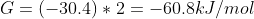. Reaction D has a delta G of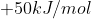; when coupled with the hydrolization of 2 moles of ATP, delta G will be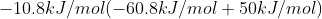. As this is negative, it is indicated that this will be a spontaneous reaction, or can proceed without input of energy.

### Example Question #1 : Understand Atp Coupling

ATP coupling describes the process through which hydrolysis of ATP can drive a __ reaction

exothermic

spontaneous

thermodynamically unfavorable

thermodynamically favorable

thermodynamically unfavorable

Explanation:

ATP coupling is the process where hydrolysis of ATP (a thermodynamically favorable, negative delta G, or spontaneous reaction) is coupled with a thermodynamically unfavorable reaction (a reaction that cannot proceed without energy input).

### Example Question #2 : Understand Atp Coupling

Which of the following is an endergonic reaction?

Catabolism

Na+/K+ pump

Hydrolysis of ATP

Cellular respiration

Na+/K+ pump

Explanation:

An endergonic reaction requires input of energy; delta G will be positive. Hydrolysis of ATP, cellular respiration, and catabolism (breakdown of a large molecule) are exergonic processes. The Na+/K+ pump is endergonic, as it requires energy. This process is coupled with ATP hydrolysis to allow it to proceed.

### Example Question #1 : Cellular Energetics

Which is true of an exergonic reaction?

The reaction will not occur spontaneously

Energy is released

The reaction will always be endothermic

Energy is required

Energy is released

Explanation:

Exergonic reactions are reactions that release energy. Endergonic reactions are reactions that require energy to proceed. Exergonic reactions can occur spontaneously, or are thermodynamically favorable. Endergonic reactions cannot occur spontaneously, or are thermodynamically unfavorable.

### Example Question #1 : Understand Atp Coupling

ATP phosphorylation (ADP+ Pi-> ATP+ H2O) is what type of reaction?

exergonic

catabolic

endergonic

spontaneous

endergonic

Explanation:

ATP hydrolysis is exergonic. ATP phosphorylation is the reverse of ATP hydrolysis, and thus is endergonic.

### Example Question #2 : Cellular Energetics

If the reaction for ATP hydrolysis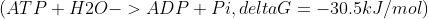is coupled with the reaction glucose+ sucrose-> fructose,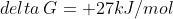, what is the net delta G?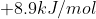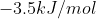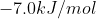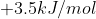Through ATP coupling, the hydrolysis of ATP can allow the second, thermodynamically unfavorable reaction to proceed.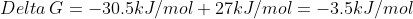. As the resulting delta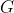is negative, this will be thermodynamically favorable.